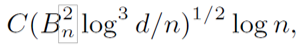# Nearly optimal central limit theorem and bootstrap approximations in high dimensions

4 March 2021

### Type

Working Paper (CWP08/21)

### DOI

In this paper, we derive new, nearly optimal bounds for the Gaussian approximation to scaled averages of n independent high-dimensional centered random vectors X1, . . . , Xn over the class of rectangles in the case when the covariance matrix of the scaled average is non-degenerate. In the case of bounded Xi’s, the implied bound for the Kolmogorov distance between the distribution of the scaled average and the Gaussian vector takes the formwhere d is the dimension of the vectors and Bn is a uniform envelope constant on components of Xi’s. This bound is sharp in terms of d and Bn, and is nearly (up to log n) sharp in terms of the sample size n. In addition, we show that similar bounds hold for the multiplier and empirical bootstrap approximations. Moreover, we establish bounds
that allow for unbounded Xi’s, formulated solely in terms of moments of Xi’s. Finally, we demonstrate that the bounds can be further improved in some special smooth and zero-skewness cases.